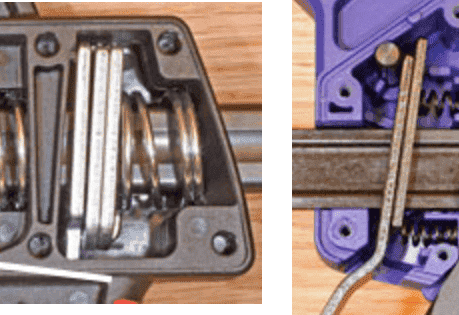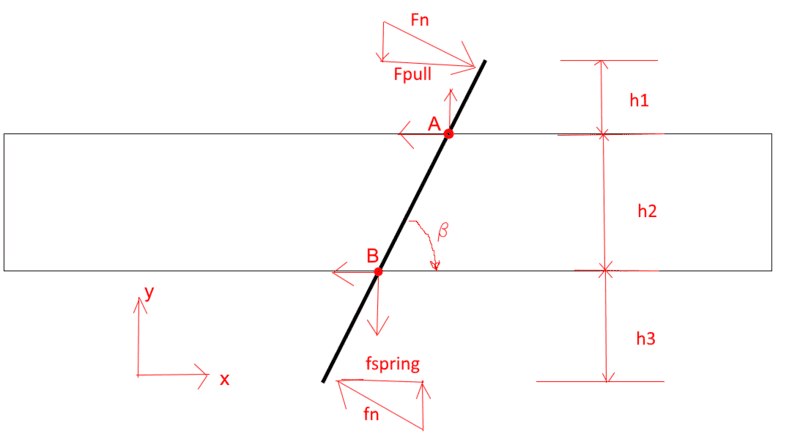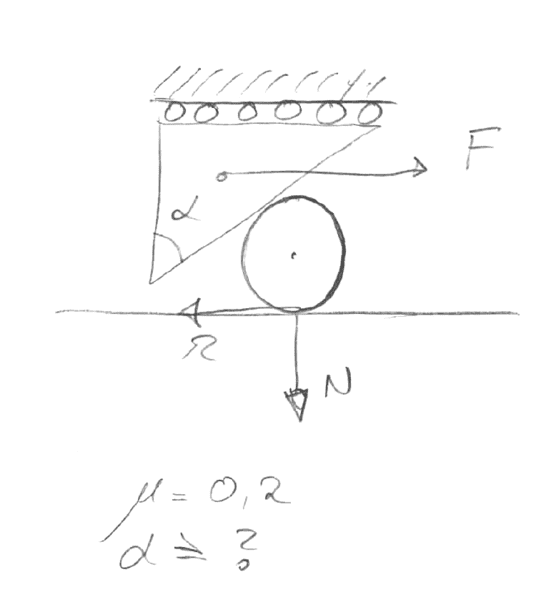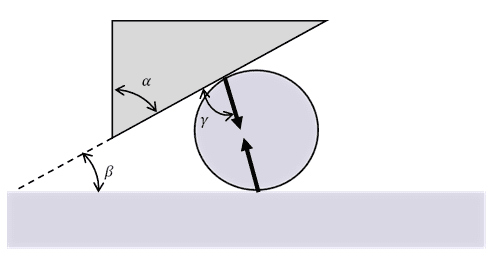# Quick clamp wedge/friction lock calculation

I am currently working on designing a linear brake/lock for a completely different purpose but it will be based on the same principle as in a quick clamp shown below. Before building a prototype I do want to make some rough calculations to check dimensions, angles etc. etc. However, I am for some reason struggling with the calculation and can't seem to get it right.

You can see my figure below. Assume no friction from applied forces Fpull and fspring (that is why they are not hypotenus)

My question is. How can I easily check whether or not the locking will hold a specific Fpull-load with given geometries and input data as shown below? I would like to create a an excel spreadsheet. Perhaps should one find at which friction it would hold instead?
h1=20
h2=40
h3=20
Fpull=7000N
friction=0,3 (should this be an unknown instead?)
Fspring=50N
Beta=5 degrees

I would greatly appreciate any feedback on this problem. It should be fairly simple I think, but I am caught in a thought loop on this.#### Attachments

jambaugh
Gold Member
The key to these sorts of locking mechanisms is that an increase in the force against the locking action will increase (up to some failure point) the locking force itself.

So in this case the springs don't have as much to do with it as does the angle of the locking plates. Notice the metal pin on the top half of your left photograph. That's your pivot point for the locking plates and it thus causes the pull on the rod to transmit a normal force on its edges due to the pivot.

Hmmm.... this one's a bit tricky but I'm going to assume that the normal forces for each side of the clamping plate is the same ##F_n = f_n## so you should see a normal force proportional to the net torque created by the two normal forces. Let's see their orthogonal separation is ##h_2 sin(\beta)## so the torque resulting from the clamping force will be ## \tau = F_n \cdot h_2 \cdot sin(\beta) ##.

Now I'm going to assume the corresponding frictional forces on each edge are likewise the same meaning the pulling force that the friction opposes is balanced and can be considered to act along the center of the rod. Thus its distance from the pivot is ## h_1+0.5h_2## (assuming ##h_1## is measured off that pivot pin) and so the torque induced by the load must likewise be ##\tau = F_{pull}\cdot (h_1+0.5 h_2)##. Equating the torques we get a relation between the angle, distances and the coefficient of friction for the clamping effect.

Note that since the pulling force is opposed by two points of friction with equal values we here have ##F_{pull} = 2\mu_{f} F_n## and thence:
$$2\mu_f\cdot (h_1 + 0.5 h_2) = h_2 \sin(\beta)$$
or
$$\mu_f \cdot (2h_1/h_2 + 1) = \sin(\beta)$$

That's my derivation here on the spot. I'd add a factor of 2 or maybe 10 for safety. I ignored the spring force but it really has a small effect. The spring is there to get the clamping plate to initially bite into the rod in order to get enough starting friction to achieve the locking action. At least that's my not-so-humble opinion.

•Andreas MH
Thank you for further insight into the problem. It absolutely makes sense that the force is out of the equation at the end.
However, by adding some numbers for testing:
Beta=5 degrees
h1=20mm
h2=40mm
This yields a required friction coefficient of as little as my=0,043. Can this really be correct?
I had two other different, more complex ways of getting to a results, and with both those solutions and same input as above I also got to a value of 0,043. But I thought that value was way too low and I refused to believe the result. What is your opinion on that?

-Andreas-

jambaugh
Gold Member
I don't think the low coefficient is that surprising. To appreciate this remember that the clamping force is being used to produce the normal force for the friction. Also you might find it helpful to your intuition to look at the actual force on the side of the slide rod given the pulling force for a given angle. Also mind that this is the "just good enough to hold" calculation. The ideal would be a significantly higher coefficient, say 5 times this calculation?

•Andreas MH
I don't think the low coefficient is that surprising. To appreciate this remember that the clamping force is being used to produce the normal force for the friction. Also you might find it helpful to your intuition to look at the actual force on the side of the slide rod given the pulling force for a given angle. Also mind that this is the "just good enough to hold" calculation. The ideal would be a significantly higher coefficient, say 5 times this calculation?

Over to a different sort of "wedging" or self-enhancing lock. How would you solve this? What would be the required angle in this particular case to ensure locking?#### Attachments

A.T.
Over to a different sort of "wedging" or self-enhancing lock. How would you solve this? What would be the required angle in this particular case to ensure locking?

View attachment 228942

Is the friction coefficient the same for both contacts of the cylinder?

jambaugh
Gold Member
Andreas, I'm not clear on your diagram. Could you elaborate a bit?

Is the friction coefficient the same for both contacts of the cylinder?

Same friction on all contact surfaces.

Andreas, I'm not clear on your diagram. Could you elaborate a bit?

I will try. The bottom line is fixed/immovable and serves as one side of a rail per say. The wedge shape is part of a trolley moving along the rail, but unable to move perpendicular to the rail(bottom line) only parallel movement is allowed. There is two friction contact surfaces wedge-cylinder and cylinder-rail. They both have the same friction coefficient for sake of simplicity.

My question is, what is the minimum angle alpha needed for the system to lock and prevent movement of the trolley with a given force F and friction my=0,2.

Hope this makes it more clear. I am trying to design a holding brake which does not jam and does not have any backlash (system will involve a spring which will be actuated by a lever, but that comes later).

-Andreas-

This is an interesting problem. My intuition (probably wrong) is that unless there is some external force applied to the roller (to the left), it will just 'roll away' from the wedge for any wedge angle. I know that this mechanism will 'work,' but I suspect that (absent the counter force) is the result of deformation and dynamic forces (roller inertia as the counter-force). Given a counter force, the mechanism 'locks' when the roller/wedge interface prevents rolling ('sliding' at that point) and the roller/rail interface prevents sliding. Or not.

jambaugh
Gold Member
Ok, Got it. Hmmm.... I'm not going to try and resolve the whole problem in this post but will demonstrate how I approach it.
With friction forces I know that the forces on and from the cylinder are not necessarily normal to the surface. I do know that, assuming we are at the point of breakdown where we have maximal holding force applied that the tangential component of the contact forces on the cylinder will be equal to the coefficient of static friction times the normal components. Finally since the cylinder is not accelerating the total force and torque on it are zero (don't know yet if I need to invoke torques). This means the applied force on the cylinder by the lower rail equals the applied force on the wedge by the cylinder. And finally the horizontal component of the force of the cylinder on the wedge must be equal and opposite to the applied force on the wedge in order to lock it.

I am cautioning myself that I should not yet assume that both points on the cylinder are simultaneously at the breakaway point with regard to static friction. In fact I seem to recall an exercise where one could cause a cylinder to roll or slide depending on the applied force but that faint memory of an example may not have the same constraints as here. Let's see... forgetting the application here imagine a cylinder in a symmetric wedge. If you "jam" the cylinder in, for certain angles it should stick due to contact friction. Depending on the degree of friction there should be other angles where the cylinder will never "stick". Obviously obtuse angles would not hold the cylinder. Since in this example there are no other forces on the cylinder we can in fact treat it as one of these examples. The cylinder doesn't know which way is "horizontal" or not it just knows it's stuck (just barely) between two planes at angles to each other. So that's were I would begin my attack on the problem.

[edit:] and my earlier caution is moot. The forces on the cylinder will be symmetric provided the coefficients of friction are equal.

jambaugh
Gold Member
Continuing the analysis. Assuming the cylinder is on the verge of breaking out of the wedge, the applied forces will have tangential component ##\mu## times the normal component. Let's call ##\beta## the complementary angle to ##\alpha## hmmm... I just realized that since the net force on the cylinder must be zero, the force due to one point of contact on the cylinder must be applied directly toward the other point of contact so the two can cancel as vectors. We automatically know the angle of the force off the normal. That's just geometry and will give us our coefficient of friction.You have an isosceles triangle with angles ##\beta,\gamma, \gamma## with ##2\gamma = 180^\circ- \beta = 180^\circ - (90^\circ - \alpha)=90^\circ + \alpha## so ##\gamma = 45^\circ+ \alpha/2##. Now the angle off the normal is the complement of ##\gamma## so is ##45^\circ - \alpha/2##.
The ratio of tangential force to normal force must then be:
$$\mu_f = \tan(45^\circ-\alpha/2)$$
Yes, I think that's right. When ##\alpha = 0## you have a 90 degree wedge and the tangent and normal forces must be equal for the friction of one face to oppose the normal force of the other. We get ##\tan(45^\circ)=1## as reasoned. When ##\alpha=90^\circ## the cylinder is between two parallel planes, no significant friction is needed to hold them there and we get ##\tan(0)=0## as expected.

So I believe this reasoning and this formula is correct. (BTW I hope you're being honest here and I've not just done your course homework for you :)

#### Attachments

•Andreas MH
Continuing the analysis. Assuming the cylinder is on the verge of breaking out of the wedge, the applied forces will have tangential component ##\mu## times the normal component. Let's call ##\beta## the complementary angle to ##\alpha## hmmm... I just realized that since the net force on the cylinder must be zero, the force due to one point of contact on the cylinder must be applied directly toward the other point of contact so the two can cancel as vectors. We automatically know the angle of the force off the normal. That's just geometry and will give us our coefficient of friction.

View attachment 229051

You have an isosceles triangle with angles ##\beta,\gamma, \gamma## with ##2\gamma = 180^\circ- \beta = 180^\circ - (90^\circ - \alpha)=90^\circ + \alpha## so ##\gamma = 45^\circ+ \alpha/2##. Now the angle off the normal is the complement of ##\gamma## so is ##45^\circ - \alpha/2##.
The ratio of tangential force to normal force must then be:
$$\mu_f = \tan(45^\circ-\alpha/2)$$
Yes, I think that's right. When ##\alpha = 0## you have a 90 degree wedge and the tangent and normal forces must be equal for the friction of one face to oppose the normal force of the other. We get ##\tan(45^\circ)=1## as reasoned. When ##\alpha=90^\circ## the cylinder is between two parallel planes, no significant friction is needed to hold them there and we get ##\tan(0)=0## as expected.

So I believe this reasoning and this formula is correct. (BTW I hope you're being honest here and I've not just done your course homework for you :)

haha, you just solved my homework :-) no, seriously, I work as a product design engineer and with a large variation of types of projects/products it is difficult for me to stay sharp on all subjects and that is why I am posting questions here. Every now and then I am in need of making preliminary calculations on mechanical concepts before making prototypes for testing of the principles and they are rarely similar. Thank you very much for helping out!

jambaugh